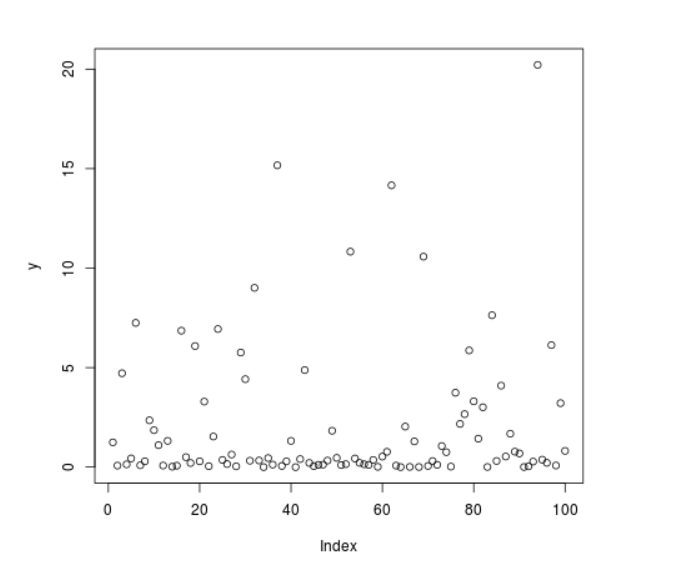# Compute Randomly Drawn Weibull Density in R Programming – rweibull() Function

• Last Updated : 25 Jun, 2020

`rweibull()` function in R Language is used to compute random density for Weibull distribution.

Syntax: rweibull(N, shape)

Parameters:
N: Sample Size
shape: Shape Parameter

Example 1:

 `# R program to compute random``# Weibull Density`` ` `# Setting seed for``# random number generation``set``.seed(``1000``)`` ` `# Set sample size``N <``-` `20`` ` `# Calling rweibull() Function``y <``-` `rweibull(N, shape ``=` `0.5``)``y`

Output:

```  1.24347372 0.07615159 4.71808321 0.13687770 0.43674779 7.24744843
 0.09171405 0.29014465 2.35173196 1.85531597 1.10250171 0.07863894
 1.31925387 0.02076093 0.07234868 6.85850214 0.50129483 0.20600265
 6.08499134 0.28943273
```

Example 2:

 `# R program to compute random``# Weibull Density`` ` `# Setting seed for``# random number generation``set``.seed(``1000``)`` ` `# Set sample size``N <``-` `100`` ` `# Calling rweibull() Function``y <``-` `rweibull(N, shape ``=` `0.5``)`` ` `# Plot a graph``plot(y)`

Output:My Personal Notes arrow_drop_up